1668488605

# What are Double Parentheses in Bash

## What are Double Parentheses in Bash

“Brackets are important in any programming language to execute the program and get the desired outputs. In bash, we use single and double parentheses to perform different tasks through a script. However, many beginners and even intermediate bash users need to learn about double parentheses. So in this tutorial, we will briefly summarize what double parentheses are in bash.”

## What are Double Parentheses in Bash

The “((..))” double parentheses are similar to the let command, which you can use to perform arithmetic calculations in a script. For example, let’s create a script that will perform various arithmetic calculations:

``````#!/bin/bash
echo "Please enter the values of A and B"
sum=\$((A+B))
echo "Addition of \$A and \$B is \$sum"

sub=\$((A-B))
echo "Subtraction of \$A and \$B is \$sub"

multiply=\$((A*B))
echo "Multiplication \$A and \$B is \$multiply"

division=\$((A/B))
echo "Division of \$A and \$B is \$division"``````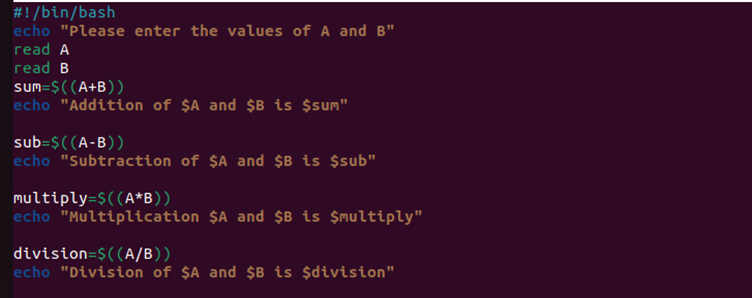Once you run the above script, the terminal will ask you to enter two numbers, and then it will perform the calculations:

Similarly, you can use the following patterns of double parentheses styles to get the required results:

``````#!/bin/bash
echo "Please enter the values of A and B"
sum=\$((A+B))
echo "Addition of \$A and \$B is \$sum"

((sub=A-B))
echo "Subtraction of \$A and \$B is \$sub"

num1=A
num2=B

((multiply=num1*num2))
echo "Multiplication \$A and \$B is \$multiply"

division=\$((num1/num2))
echo "Division of \$A and \$B is \$division"``````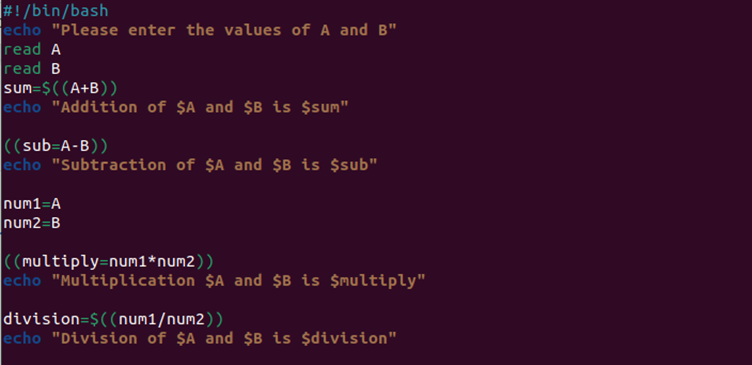This script provides the same result as the previous one: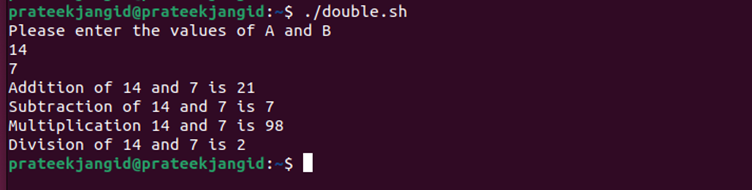You can also use the [[ rather than [  because it is an advanced type that offers a ton of enhancements such as:

• The [[ can efficiently handle the empty strings and some strings with the whitespace.
• You can use || and && logical operators with [[ but not [ because single [ can’t pass || and && operator as a command-line argument.

## Wrapping Up

This was all about the double parentheses in bash, which you can try to enhance the arithmetic calculations easily. We have explained various types of examples to perform arithmetic calculations by adding double parentheses. Bash contains a ton of concepts that you can learn to become a bash expert. So make sure you check out Linuxhint to read various tutorials of bash.

Original article source at: https://linuxhint.com/

## Buddha Community1668753367

## What is Double Parentheses in Bash?

“Brackets are important in any programming language to execute the program and get the desired outputs. In bash, we use single and double parentheses to perform different tasks through a script. However, many beginners and even intermediate bash users need to learn about double parentheses. So in this tutorial, we will briefly summarize what double parentheses are in bash.”

## What are Double Parentheses in Bash

The “((..))” double parentheses are similar to the let command, which you can use to perform arithmetic calculations in a script. For example, let’s create a script that will perform various arithmetic calculations:

``````#!/bin/bash
echo "Please enter the values of A and B"
sum=\$((A+B))
echo "Addition of \$A and \$B is \$sum"

sub=\$((A-B))
echo "Subtraction of \$A and \$B is \$sub"

multiply=\$((A*B))
echo "Multiplication \$A and \$B is \$multiply"

division=\$((A/B))
echo "Division of \$A and \$B is \$division"``````Once you run the above script, the terminal will ask you to enter two numbers, and then it will perform the calculations: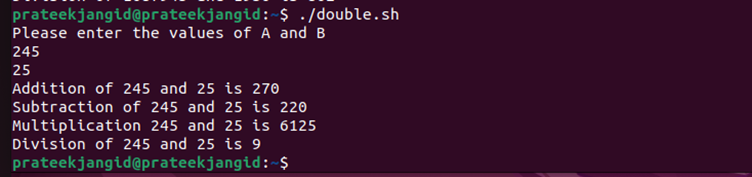Similarly, you can use the following patterns of double parentheses styles to get the required results:

``````#!/bin/bash
echo "Please enter the values of A and B"
sum=\$((A+B))
echo "Addition of \$A and \$B is \$sum"

((sub=A-B))
echo "Subtraction of \$A and \$B is \$sub"

num1=A
num2=B

((multiply=num1*num2))
echo "Multiplication \$A and \$B is \$multiply"

division=\$((num1/num2))
echo "Division of \$A and \$B is \$division"``````This script provides the same result as the previous one:You can also use the [[ rather than [  because it is an advanced type that offers a ton of enhancements such as:

• The [[ can efficiently handle the empty strings and some strings with the whitespace.
• You can use || and && logical operators with [[ but not [ because single [ can’t pass || and && operator as a command-line argument.

## Wrapping Up

This was all about the double parentheses in bash, which you can try to enhance the arithmetic calculations easily. We have explained various types of examples to perform arithmetic calculations by adding double parentheses. Bash contains a ton of concepts that you can learn to become a bash expert. So make sure you check out Linuxhint to read various tutorials of bash.

Original article source at: https://linuxhint.com/1668488605

## What are Double Parentheses in Bash

“Brackets are important in any programming language to execute the program and get the desired outputs. In bash, we use single and double parentheses to perform different tasks through a script. However, many beginners and even intermediate bash users need to learn about double parentheses. So in this tutorial, we will briefly summarize what double parentheses are in bash.”

## What are Double Parentheses in Bash

The “((..))” double parentheses are similar to the let command, which you can use to perform arithmetic calculations in a script. For example, let’s create a script that will perform various arithmetic calculations:

``````#!/bin/bash
echo "Please enter the values of A and B"
sum=\$((A+B))
echo "Addition of \$A and \$B is \$sum"

sub=\$((A-B))
echo "Subtraction of \$A and \$B is \$sub"

multiply=\$((A*B))
echo "Multiplication \$A and \$B is \$multiply"

division=\$((A/B))
echo "Division of \$A and \$B is \$division"``````Once you run the above script, the terminal will ask you to enter two numbers, and then it will perform the calculations:

Similarly, you can use the following patterns of double parentheses styles to get the required results:

``````#!/bin/bash
echo "Please enter the values of A and B"
sum=\$((A+B))
echo "Addition of \$A and \$B is \$sum"

((sub=A-B))
echo "Subtraction of \$A and \$B is \$sub"

num1=A
num2=B

((multiply=num1*num2))
echo "Multiplication \$A and \$B is \$multiply"

division=\$((num1/num2))
echo "Division of \$A and \$B is \$division"``````This script provides the same result as the previous one:You can also use the [[ rather than [  because it is an advanced type that offers a ton of enhancements such as:

• The [[ can efficiently handle the empty strings and some strings with the whitespace.
• You can use || and && logical operators with [[ but not [ because single [ can’t pass || and && operator as a command-line argument.

## Wrapping Up

This was all about the double parentheses in bash, which you can try to enhance the arithmetic calculations easily. We have explained various types of examples to perform arithmetic calculations by adding double parentheses. Bash contains a ton of concepts that you can learn to become a bash expert. So make sure you check out Linuxhint to read various tutorials of bash.

Original article source at: https://linuxhint.com/1595303760

## Bash Select (Make Menus) and Bash select Example

In this tutorial, we will cover the basics of the `select` construct in Bash.

The `select` construct allows you to generate menus.

## Bash `select` Construct

The `select` construct generates a menu from a list of items. It has almost the same syntax as the `[for](https://linuxize.com/post/bash-for-loop/)` loop:

``````select ITEM in [LIST]
do
[COMMANDS]
done
``````

Copy

The `[LIST]` can be a series of strings separated by spaces, a range of numbers, output of a command, an array, and so on. A custom prompt for the `select` construct can be set using the `PS3` environment variable.

When the `select` construct is invoked, each item from the list is printed on the screen (standard error), preceded with a number.

If the user enters a number that corresponds to the number of one of the displayed items, then the value of `[ITEM]` is set to that item. The value of the selected item is stored in the variable `REPLY`. Otherwise, if the user input is empty, the prompt and the menu list are displayed again.

The`select`loop will continue to run and prompt for user input until the`[break](https://linuxize.com/post/bash-break-continue/)`command is executed.To demonstrate how the `select` construct works, let’s take a look at the following simple example:

``````PS3="Enter a number: "

select character in Sheldon Leonard Penny Howard Raj
do
echo "Selected character: \$character"
done
``````

#bash #bash select construct #bash select1671787740

## What is a Bash Script? | How to Write a Bash Script

In this Bash Script article, we will explore What is a Bash Script? | How to Write a Bash Script. Discover the basics of bash scripting and learn how to write a bash script. A shell is a kind of computer program that presents an interface to the operating system. The name shell was derived from the fact that it is the outermost layer around the operating system.

To manage the interaction between users and the operating system, the shell prompts for an input and then interprets that input for the operating system before handling any outputs it receives. This input may be provided via a command line interface (CLI) or graphical user interface (GUI), depending on the operation and the role the computer takes on.

Check out our What is Shell? article to delve deeper into what a shell is and how it can help you.

Essentially, a shell enables users and other programs to communicate directly with an operating system’s components.

## A Primer on Shell Scripting

Shell scripting is an important yet easy-to-learn (even if you are not from a programming background) part of communicating with a computer’s operating system. By scripting, we are referring to a sequence of shell and operating system commands that are written and then stored in a file: typical operations performed by shell scripts include tasks such as file manipulation, program execution, and printing text.

Learning to perform this simple procedure can save developers a significant amount of development time as certain commands will not have to be manually written repeatedly – it is even possible to schedule scripts to be executed automatically.

There are several different shells available to Unix and Linux users. For the remainder of this tutorial, we will focus specifically on the Bourne Again SHell (bash), which is an enhanced version of the Bourne shell and is one of the most common command line interfaces for Unix-based operating systems (including Linux).

## What is a Bash Script?

Bash is a Unix command line interface responsible for interacting with a computer's operating system. Similarly to how movie scripts inform actors of what actions to take, a bash script tells the bash shell what to do. Thus, a bash script is a useful way to group commands to create a program

Any command you could execute directly from the command line can be put into a bash script, and you could expect it to do the same actions as it would from the command line. Alternatively, any command you enter into your bash script could be run directly from the command line, and the result should be the same. In other words, if you know how to interact with your computer from the command line, you already know a fair bit about bash scripting.

One of the main differences is a bash script is a plain text file that is stored with a `.sh` extension by convention (they can still run without the `.sh` extension).

Another distinction of bash scripts is the “shebang” that occurs on the first line of all scripts. Essentially, the shebang is an absolute path to the bash interpreter made up of a combination of bash “#” and bang “!” followed by the bash shell path. It is used to tell the Linux operating system which interpreter to use to parse the file.

## How to Write a Bash Script

We are going to build a simple “Hello World” bash script example to get you started with bash scripting. If you wish to delve deeper into bash scripting, check out Introduction to Bash Scripting course.

### Step 1: Create a new plain text file

The first step is to create a new plain text file. You can do this by running the following command from your command prompt:

``notepad hello_world.sh``

Note: Executing this command may bring a prompt asking you if you would like to create a new file; select “Yes.”

### Step 2: Specifying the interpreter

On the first line of our script, we must specify which interpreter we would like to use to parse our script. In this scenario, it is Bash. Thus, we must put the shebang in the first line of our script.

``#!/bin/bash``

### Step 3: Implement commands

The purpose of our bash script is to print “Hello World!” To perform this task, move to a new line and use the echo command followed by the string we would like to print.

``echo "Hello World!" ``

Once complete, save the file and open up your Git Bash.

Next, run the following command:

``bash hello_world.sh``

This should output “Hello World!” as seen in Figure 1.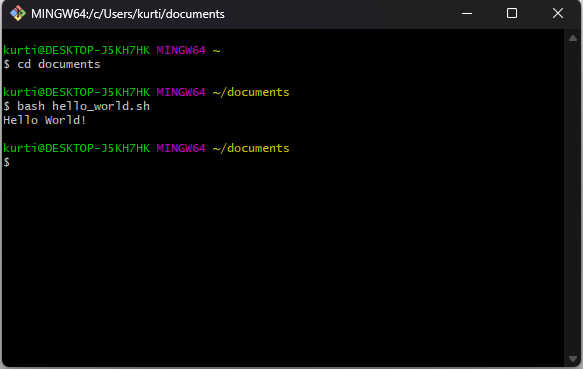Figure 1: The output of our Bash script

## How to Capture User Input in Bash Scripts

Now you know how to write a simple “Hello World!” script. Let’s take a look at how we could use bash to write scripts that require input from the user. In this example, we will create an activity generator, which will ask the user for their name and then choose a random activity to invite the user to participate in from an array of activities.

The steps to create the file are the same as in How to Write a Bash Script – the difference are the commands we enter into our bash script.

1. Create a new plain text file from the command prompt using notepad.
``notepad generate-activity.sh ``

2.    Specify the bash interpreter by entering the shebang in the first line of the script.

``#!/bin/bash``

3.    Write the commands to run the script and then save the file.

Now when we call our bash script from the terminal, we should expect to see the same output as in Figure 2.

``````#!/bin/bash

# Greet user and request their name
echo "The activity generator"

# Create an array of activities
activity="Football"
activity="Table Tennis"
activity="8 Ball Pool"
activity="PS5"
activity="Blackjack"

array_length=\${#activity[@]} # Store the length of the array
index=\$((\$RANDOM % \$array_length)) # Randomly select an index from 0 to array_length

# Invite the user to join you participate in an activity
echo "Hi" \$name, "would you like to play" \${activity[\$index]}"?"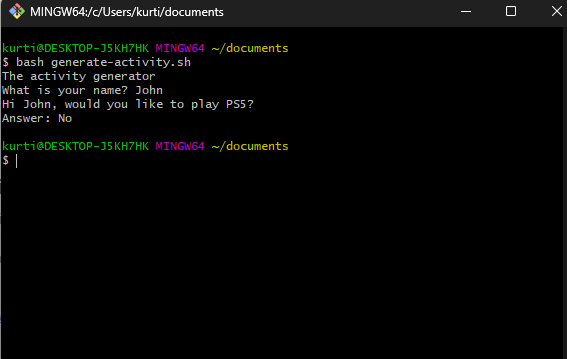Figure 2: Demonstrating the generate-activity.sh script.

## How to Create a Function in a Bash Script

In programming, functions are used to bundle a set of repeated instructions together – learn more about Functions in Python. The purpose of writing functions is to avoid writing the same code constantly, and it also makes your code more readable.

A bash function may be considered a typical function: a set of commands that can be called several times. However, bash functions are slightly more limited when compared to the majority of programming languages (i.e., bash functions do not allow you to return a value when called).

Before we create a bash script using a function, let’s take a look at the syntax for declaring a bash function. We can define a function by writing a function name, followed by parentheses and curly braces for the function's body – this is the most common way to write a bash function. Here is it looks in code:

``````# Most common way to write a bash function.
example_function () {
commands
{``````

The other way to format a bash function is to begin the line with the reserved bash keyword function, then the function name and curly braces for the function body – we do not need the parentheses.

``````# Another way to write a bash function.
function example_function {
commands
{``````

Defining the function will not execute the commands. To invoke a bash function, you must call the function name, as follows:

``````#!/bin/bash

# A hello world bash function
hello_world () {
echo "Hello World!"
}

# Invoking the hello_world function
hello_world``````

Above, we created a  hello_world function that prints “Hello World!” when the bash script is run.

### Passing arguments to bash functions

Arguments are variables used in a specific function. In bash, we can not pass arguments to functions like you would in high-level programming languages such as Python, Julia, etc. Instead, bash functions have their own command line argument and we can pass parameters to a function by placing them after a call to the function name – separated by a space if there are multiple arguments.

In essence, function arguments are treated as positional parameters and shell variables (\$1, \$2, ..., \$n) are used to access arguments passed to the function.

``````#!/bin/bash
greet () {
echo "Hello \$1 \$2"
}

greet "John" "Doe" ``````

In the bash script above, we passed two arguments, John and Doe,  to our greet function. When the bash script is run now, we should see the following output:

``Hello John Doe``

## Writing “If” Statements in Bash

“If” statements are conditional statements used in programming languages. If a statement is proven to be true, then a condition would run. This functionality enables us to determine the circumstances under which a certain piece of code shall be executed.

In bash, we start the conditional with an if statement and end it with fi (if spelled backward). Here is an example bash script with if conditionals:

``````#!/bin/bash

read -p "Give me a number: " number
if [ \$number -gt 10 ];
then
echo "Your number is greater than 10."
fi``````

In the bash script above, the user is asked to provide a number. If the number provided by the user is greater than 10, then “Your number is greater than 10” will be outputted to the terminal, but if it is not, the program will end. Figure 3 is an example of us passing a number larger than 10.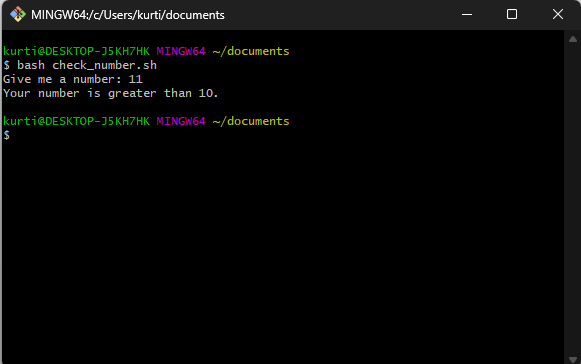Figure 3: Using “if” statements in Bash scripts

## Wrap-up

Bash scripts are a useful way to elegantly group commands together. You could leverage the power of a bash script for a number of use cases, such as executing a shell command, running multiple commands together, customizing administrative tasks, performing task automation, and more.

By following the steps provided in the tutorial above, you now know how to create a simple script to print “Hello World!” using Bash.

Original article sourced at: https://www.datacamp.com

#bash #Bash-Script1594881960

## Bash break and continue

Loops allow you to run one or more commands multiple times until a certain condition is met. However, sometimes you may need to alter the flow of the loop and terminate the loop or only the current iteration.

In Bash, `break` and `continue` statements allows you to control the loop execution.

## Bash `break` Statement

The `break` statement terminates the current loop and passes program control to the command that follows the terminated loop. It is used to exit from a `for``while``[until](https://linuxize.com/post/bash-until-loop/)`, or `select` loop. s The syntax of the `break` statement takes the following form:

``````break [n]
``````

Copy

`[n]` is an optional argument and must be greater than or equal to 1. When `[n]` is provided, the n-th enclosing loop is exited. `break 1` is equivalent to `break`.

To better understand how to use the `break` statement, let’s take a look at the following examples.

In the script below, the execution of the `[while](https://linuxize.com/post/bash-while-loop/)` loop will be interrupted once the current iterated item is equal to `2`:

``````i=0

while [[ \$i -lt 5 ]]
do
echo "Number: \$i"
((i++))
if [[ \$i -eq 2 ]]; then
break
fi
done

echo 'All Done!'
``````

Copy

``````Number: 0
Number: 1
All Done!
``````

#bash #bash break #bash continue statement